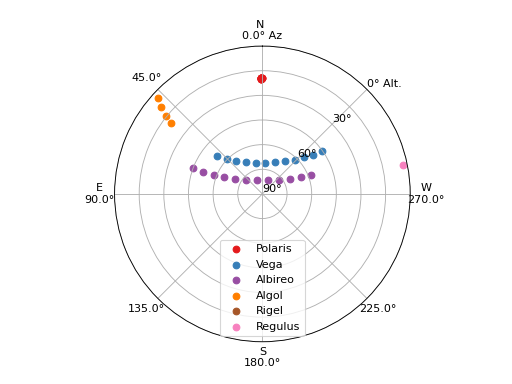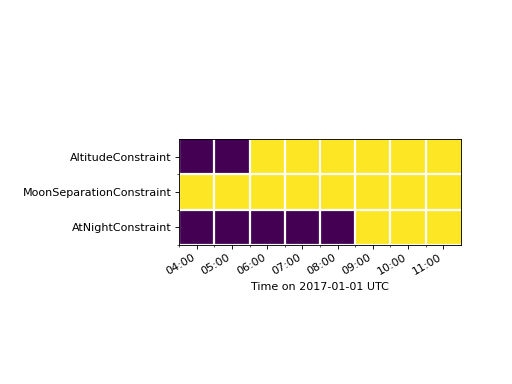# Defining Observing Constraints¶

## Introduction to Built-In Constraints¶

Frequently, we have a long list of targets that we want to observe, and we need to know which ones are observable given a set of constraints imposed on our observations by a wide range of limitations. For example, your telescope may only point over a limited range of altitudes, your targets are only useful in a range of airmasses, and they must be separated from the moon by some large angle. The `constraints` module is here to help!

Say we’re planning to observe from Subaru Observatory in Hawaii on August 1, 2015 from 06:00-12:00 UTC. First, let’s set up an `Observer` object:

```from astroplan import Observer, FixedTarget
from astropy.time import Time
subaru = Observer.at_site("Subaru")
time_range = Time(["2015-08-01 06:00", "2015-08-01 12:00"])
```

We’re keeping a list of targets in a text file called `targets.txt`, which looks like this:

```# name ra_degrees dec_degrees
Polaris 37.95456067 89.26410897
Vega 279.234734787 38.783688956
Albireo 292.68033548 27.959680072
Algol 47.042218553 40.955646675
Rigel 78.634467067 -8.201638365
Regulus 152.092962438 11.967208776
```

We’ll read in this list of targets using `astropy.table`, and create a list of `FixedTarget` objects out of them:

```# Read in the table of targets
from astropy.table import Table

# Create astroplan.FixedTarget objects for each one in the table
from astropy.coordinates import SkyCoord
import astropy.units as u
targets = [FixedTarget(coord=SkyCoord(ra=ra*u.deg, dec=dec*u.deg), name=name)
for name, ra, dec in target_table]
```

We will build a bulleted list of our constraints first, then implement them in code below.

• Our observations with Subaru can only occur between altitudes of ~10-80 degrees, which we can define using the `AltitudeConstraint` class.

• We place an upper limit on the airmass of each target during observations using the `AirmassConstraint` class.

• Since we’re optical observers, we only want to observe targets at night, so we’ll also call the `AtNightConstraint` class. We’re not terribly worried about sky brightness for these bright stars, so we’ll define “night” times as those between civil twilights by using the class method `twilight_civil`:

```from astroplan import (AltitudeConstraint, AirmassConstraint,
AtNightConstraint)
constraints = [AltitudeConstraint(10*u.deg, 80*u.deg),
AirmassConstraint(5), AtNightConstraint.twilight_civil()]
```

This list of constraints can now be applied to our target list to determine:

• whether the targets are observable given the constraints at any times in the time range, using `is_observable`,

• whether the targets are observable given the constraints at all times in the time range, using `is_always_observable`

• during what months the targets are ever observable given the constraints, using `months_observable`:

```from astroplan import is_observable, is_always_observable, months_observable
# Are targets *ever* observable in the time range?
ever_observable = is_observable(constraints, subaru, targets, time_range=time_range)

# Are targets *always* observable in the time range?
always_observable = is_always_observable(constraints, subaru, targets, time_range=time_range)

# During what months are the targets ever observable?
best_months = months_observable(constraints, subaru, targets, time_range)
```

The `is_observable` and `is_always_observable` functions will return boolean arrays which tell you whether or not each target is observable given your constraints. Let’s print these results in tabular form:

```>>> from astropy.table import Table
>>> import numpy as np
>>> observability_table = Table()
>>> observability_table['targets'] = [target.name for target in targets]
>>> observability_table['ever_observable'] = ever_observable
>>> observability_table['always_observable'] = always_observable
>>> print(observability_table)
<Table length=6>
targets ever_observable always_observable
str7        bool             bool
------- --------------- -----------------
Polaris            True              True
Vega            True              True
Albireo            True             False
Algol            True             False
Rigel           False             False
Regulus           False             False
```

Now we can see which targets are observable! You can also use the `observability_table` method to do the same calculations and store the results in a table, all in one step:

```>>> from astroplan import observability_table
>>> table = observability_table(constraints, subaru, targets, time_range=time_range)
>>> print(table)
target name ever observable always observable fraction of time observable
----------- --------------- ----------------- ---------------------------
Polaris            True              True                         1.0
Vega            True              True                         1.0
Albireo            True             False              0.833333333333
Algol            True             False              0.166666666667
Rigel           False             False                         0.0
Regulus           False             False                         0.0
```

Let’s sanity-check these results using `plot_sky` to plot the positions of the targets throughout the time range:We can see that Vega is in the sweet spot in altitude and azimuth for this time range and is always observable. Albireo is not always observable given these criteria because it rises above 80 degrees altitude. Polaris hardly moves and is therefore always observable, and Algol starts out observable but sets below the lower altitude limit, and then the airmass limit. Rigel and Regulus never rise above those limits within the time range.

## Visualizing Constraints¶

Suppose an observer is planning to observe low-mass stars in Praesepe in the optical and infrared from the W.M. Keck Observatory. The observing constraints require all observations to occur (i) between astronomical twilights; (ii) while the Moon is separated from Praesepe by at least 45 degrees; and (iii) while Praesepe is above the lower elevation limit of Keck I, about 33 degrees. These observing constraints can be specified with the `AtNightConstraint`, `MoonSeparationConstraint`, and `AltitudeConstraint` objects, like this:

We can evaluate the constraints at one hour intervals in a loop, to create an observability grid like so:

This kind of grid can be useful for visualizing what’s happening under-the-hood when you use `is_observable` or `is_always_observable`. Click the link below for the source code to produce the observability grid shown below. Dark squares represent times when the observing constraint is not satisfied.## User-Defined Constraints¶

There are many possible constraints that you could find useful which have not been implemented (yet) in astroplan. This example will walk you through creating your own constraint which will be compatible with the tools in the `constraints` module.

We will begin by defining an observer at Subaru and reading the text file of stellar coordinates defined in the example above:

```from astroplan import Observer, FixedTarget
from astropy.time import Time
subaru = Observer.at_site("Subaru")
time_range = Time(["2015-08-01 06:00", "2015-08-01 12:00"])

# Read in the table of targets
from astropy.io import ascii

# Create astroplan.FixedTarget objects for each one in the table
from astropy.coordinates import SkyCoord
import astropy.units as u
targets = [FixedTarget(coord=SkyCoord(ra=ra*u.deg, dec=dec*u.deg), name=name)
for name, ra, dec in target_table]
```

In the previous section, you may have noticed that constraints are assembled by making a list of calls to the initializers for classes like `AltitudeConstraint` and `AirmassConstraint`. Each of those constraint classes is subclassed from the abstract `Constraint` class, and the custom constraint that we’re going to write must be as well.

In this example, let’s design our constraint to ensure that all targets must be within some angular separation from Vega – we’ll call it `VegaSeparationConstraint`. Two methods, `__init__` and `compute_constraint` must be written for our constraint to work:

• The `__init__` method will accept the minimum and maximum acceptable separations a target could have from Vega.

• We’ll also define a method `compute_constraints` which takes three arguments: a `Time` or array of times to test, an `Observer` object, and some targets (a `SkyCoord` object representing a single target or a list of targets). `compute_constraints` will return an array of booleans that describe whether or not each target meets the constraints. The super class `Constraint` has a `__call__` method which will run your custom class’s `compute_constraints` method when you check if a target is observable using `is_observable` or `is_always_observable`. This `__call__` method also checks the arguments, converting single `FixedTarget` or lists of `FixedTarget` objects into an `SkyCoord` object. The `__call__` method ensures the returned array of booleans is the correct shape, so `compute_constraints` should not normally be called directly - use the `__call__` method instead.

• We also want to provide the option of having the constraint output a non-boolean score. Where being closer to the minimum separation returns a higher score than being closer to the maximum separation.

Here’s our `VegaSeparationConstraint` implementation:

```from astroplan import Constraint, is_observable, min_best_rescale
from astropy.coordinates import Angle
import astropy.units as u

class VegaSeparationConstraint(Constraint):
"""
Constraint the separation from Vega
"""
def __init__(self, min=None, max=None, boolean_constraint=True):
"""
min : `~astropy.units.Quantity` or `None` (optional)
Minimum acceptable separation between Vega and target. `None`
indicates no limit.
max : `~astropy.units.Quantity` or `None` (optional)
Minimum acceptable separation between Vega and target. `None`
indicates no limit.
"""
self.min = min if min is not None else 0*u.deg
self.max = max if max is not None else 180*u.deg
self.boolean_constraint = boolean_constraint

def compute_constraint(self, times, observer, targets):

vega = SkyCoord(ra=279.23473479*u.deg, dec=38.78368896*u.deg)

# Calculate separation between target and vega
# Targets are automatically converted to SkyCoord objects
# by __call__ before compute_constraint is called.
vega_separation = vega.separation(targets)

if self.boolean_constraint:
mask = ((self.min < vega_separation) & (vega_separation < self.max))

# if we want to return a non-boolean score
else:
# rescale the vega_separation values so that they become
# scores between zero and one
rescale = min_best_rescale(vega_separation, self.min,
self.max, less_than_min=0)
return rescale
```

Then as in the earlier example, we can call our constraint:

```>>> constraints = [VegaSeparationConstraint(min=5*u.deg, max=30*u.deg)]
>>> observability = is_observable(constraints, subaru, targets,
...                               time_range=time_range)
>>> print(observability)
[False False  True False False False]
```

The resulting list of booleans indicates that the only target separated by 5 and 30 degrees from Vega is Albireo. Following this pattern, you can design arbitrarily complex criteria for constraints.

By default, calling a constraint will try to broadcast the time and target arrays against each other, and raise a `ValueError` if this is not possible. To see the (target x time) array for the constraint, there is an optional `grid_times_targets` argument. Here we find the (target x time) array for the non-boolean score:

```>>> constraint = VegaSeparationConstraint(min=5*u.deg, max=30*u.deg,
...                                       boolean_constraint=False)
>>> print(constraint(subaru, targets, time_range=time_range,
...                  grid_times_targets=True))
[[ 0.          0.          0.          0.          0.          0.          0.
0.          0.          0.          0.          0.        ]
[ 0.          0.          0.          0.          0.          0.          0.
0.          0.          0.          0.          0.        ]
[ 0.57748686  0.57748686  0.57748686  0.57748686  0.57748686  0.57748686
0.57748686  0.57748686  0.57748686  0.57748686  0.57748686  0.57748686]
[ 0.          0.          0.          0.          0.          0.          0.
0.          0.          0.          0.          0.        ]
[ 0.          0.          0.          0.          0.          0.          0.
0.          0.          0.          0.          0.        ]
[ 0.          0.          0.          0.          0.          0.          0.
0.          0.          0.          0.          0.        ]]
```

The score of .5775 for Albireo indicates that it is slightly closer to the 5 degree minimum than to the 30 degree maximum.